# Now this baffles me.

I have a simple question. Does there exists a finite constant ${\alpha}_{0}>0$, Such that the sequence $\lbrace {a}_{n} \rbrace_{n=0}$, defined by the iteration :

${a}_{n} = {a}_{n-1}+\alpha\sin({a}_{n-1})$

Not converges to any single finite value for ${a}_{0}=1 , \alpha > {\alpha}_{0}$

What inspires me to ask this is the analysis I have done on my laptop :

What I did is that for different values of $\alpha$ , I calculated $n$ such that ${a}_{n}$ gets within $0.01 \%$ the value of $\pi$. I got this :

$\alpha = 1.99 , n=622$

$\alpha = 1.991 , n=686$

$\alpha = 1.992 , n=766$

$\alpha = 1.993 , n=867$

$\alpha = 1.994 , n=1000$

$\alpha = 1.995 , n=1183$

$\alpha = 1.996 , n=1453$

$\alpha = 1.997 , n=1893$

$\alpha = 1.998 , n=2743$

$\alpha = 1.999 , n=5150$

And the last one is :

$\alpha = 2 , n=15198162$

Also when I tried for $\alpha = 2.001$ , it just won't show any value for half an hour.

It is very weird as rate of converge gets immediately slow and I believe convergence must stop at some value of $\alpha$.

I don't know can someone do a computer analysis of this, or tell me the maths of the underlying situation.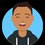Note by Ronak Agarwal
6 years, 4 months ago

This discussion board is a place to discuss our Daily Challenges and the math and science related to those challenges. Explanations are more than just a solution — they should explain the steps and thinking strategies that you used to obtain the solution. Comments should further the discussion of math and science.

When posting on Brilliant:

• Use the emojis to react to an explanation, whether you're congratulating a job well done , or just really confused .
• Ask specific questions about the challenge or the steps in somebody's explanation. Well-posed questions can add a lot to the discussion, but posting "I don't understand!" doesn't help anyone.
• Try to contribute something new to the discussion, whether it is an extension, generalization or other idea related to the challenge.

MarkdownAppears as
*italics* or _italics_ italics
**bold** or __bold__ bold
- bulleted- list
• bulleted
• list
1. numbered2. list
1. numbered
2. list
Note: you must add a full line of space before and after lists for them to show up correctly
paragraph 1paragraph 2

paragraph 1

paragraph 2

[example link](https://brilliant.org)example link
> This is a quote
This is a quote
    # I indented these lines
# 4 spaces, and now they show
# up as a code block.

print "hello world"
# I indented these lines
# 4 spaces, and now they show
# up as a code block.

print "hello world"
MathAppears as
Remember to wrap math in $$ ... $$ or $ ... $ to ensure proper formatting.
2 \times 3 $2 \times 3$
2^{34} $2^{34}$
a_{i-1} $a_{i-1}$
\frac{2}{3} $\frac{2}{3}$
\sqrt{2} $\sqrt{2}$
\sum_{i=1}^3 $\sum_{i=1}^3$
\sin \theta $\sin \theta$
\boxed{123} $\boxed{123}$

Sort by:

Here are my thoughts,

consider newtons approximation of root, let us assume that the iteration is actually of the form

${ a }_{ n-1 }-\frac { y(a_{n-1} }{ y'(a_{n-1}) }$=$a_{n})$

then comparing we require

$-\alpha \frac { y' }{ y } =cosec(x)\\$

$y=(cot(x)+cosec(x))^{ \frac { 1 }{ \alpha } }$

now after sufficient iterations, newtons method takes us to the closest root,

let us see where our expression tends to go as $x \rightarrow \pi$

$\frac { 1+cos(x) }{ sin(x) } \quad =\quad \frac { 2cos(\frac { x }{ 2 } ) }{ 2sin(\frac { x }{ 2 } ) } \simeq 0\quad for\quad x\quad close\quad to\quad \pi$

as long as $\alpha \geq 0$

hence i think it should converge for all values of $\alpha$ given $a_{0} = 1$ to $\pi$

also the rate at which we reach the root may be affected however by alpha,

as , alpha tends to $\infty$ , the whole equation tends to 1, and hence it approaches 0 perhaps more slowly and hence you see the result (not very sure of this)

you should also see raghav's method in the post where a similar question is asked with $\alpha =1$

- 6 years, 4 months ago

But this is just amazing at $\alpha=2$ , the convergence rate goes awfully slow.

- 6 years, 4 months ago

$\left(\frac{1}{\tan \left(x\right)}+\frac{1}{\sin \left(x\right)}\right)^{\frac{1}{t}}$

type this in desmos and adjust the parameter 't', you will see for yourself why it is so

https://www.desmos.com/calculator

- 6 years, 4 months ago

I have done this in fooplot , but I can't see anything that interests me or helps me in understanding this situation. By the way today OCSC-2015 list will be released(wow)

- 6 years, 4 months ago

no bro , see you will observe that when t=0.01, the graph has an almost horizontal tangent near pi and hence falls very fast to 0, at t=2, you can see that it has a near vertical tangent at x=pi meaning it doesnt fall so slow and just falls to 0 at x=pi

and yes i know and i am very afraid, really afraid, just hope to qualify

- 6 years, 4 months ago

I think we should check the conditions where newton's raphson's methos fails.

- 6 years, 4 months ago

Aah! @Ronak Agarwal I found this too! Before I posted my question, I put this in c++ and the thing failed to converge as soon as $\alpha$ became $>2$. And I think I know what's happening.

From what I saw in my program, the value of $a_n$ when $\alpha>2$ as $n\to \infty$ seems to be shuttling between two values. What may be happening is that the value overshoots $\pi$ then becomes less than $\pi$ and so on. It might be possible that for some value $a>\pi,b<\pi$, $b=a+\alpha sin(a)$ and $a=b+\alpha sin(b)$. Thus giving us an infinite non convergent loop. And yes, wolfram alpha agrees with me: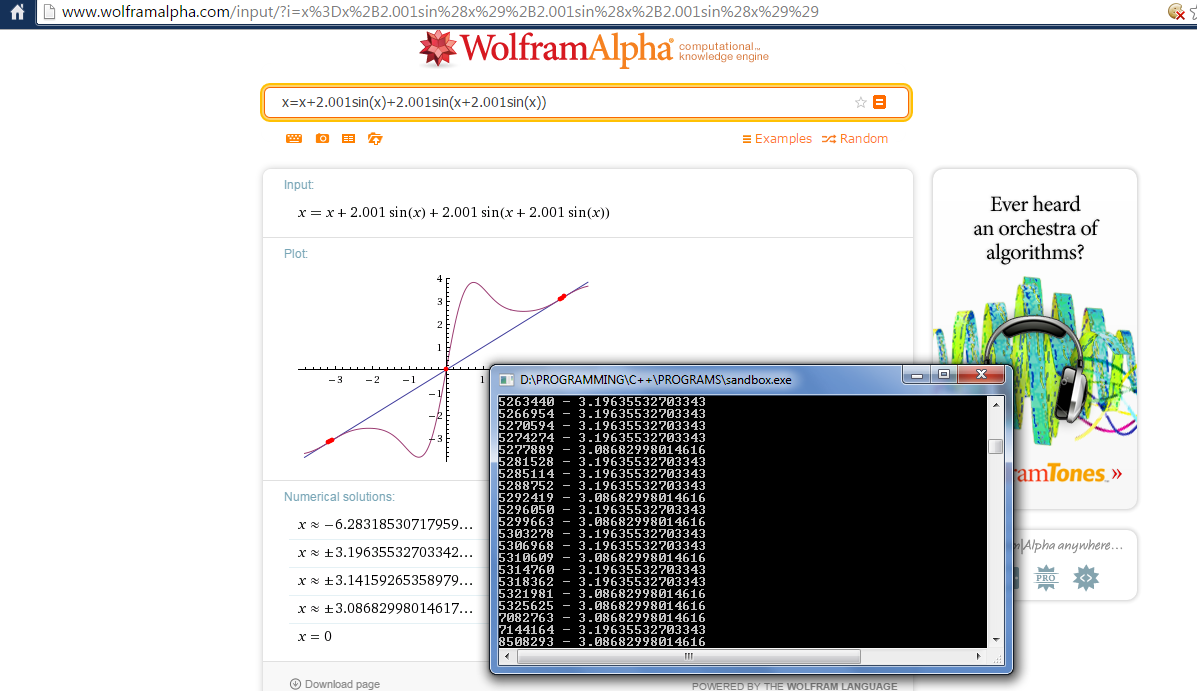I put in $x=x+2.001sin(x)+2.001sin(x+2.001sin(x))$

- 6 years, 4 months ago

@Raghav Vaidyanathan , guess what I entered the same thing into wolfram, thought exactly the same way and as I am typing this, I have tab of wolfram-alpha currently opened in which I entered EXACTLY the same thing you have entered, I figured out what is happening it is oscillating between two values, the two values I found by putting the same expression in wolfram-alpha, I love mathematical programming.

I was intending to put the same snapshot before I read your comment.

- 6 years, 4 months ago

Great! Notice that the value might have converged to pi if the initial value of $a_0$ was more than the infinite loop root value. (or so I think)

- 6 years, 4 months ago

Guess what, it again sticks to it's two-value cycle , check this Wolfram Alpha Query

- 6 years, 4 months ago

- 6 years, 4 months ago

Interestingly when $\alpha$ increases, our iteration begins to oscillate between cycles of increasing length.

For example for $\alpha=3$, it begin to oscillate between $3$ values, and so on.

- 6 years, 4 months ago

I was just studying point of inflection for board exam... God! this is interesting. Okay, so I took our equation and tried to find the conditions for which we have real roots (other than $\pi$) for $x=x+\alpha sin(x)+\alpha sin(x+\alpha sin(x))$. After simplification:

$f(x)=-(\pi -x)+(\alpha /2)sin(x)=0$)

Now we get to the interesting part. This curve is symmetric about the point $(\pi,0)$similar to the way $x^3$ is symmetric about $(0,0)$.

$f^{'}(x)=1+(\alpha /2)cos(x)$

Observe the above equation. When $\alpha /2=1$, $(\pi,0)$ is a point of inflection.

Now if we increase $\alpha /2$ to more than $1$.

$\Rightarrow f^{'}(\pi)<0$ and also$f(\pi)=0$

But there are roots for $f^{'}(x)$ on either side of $\pi$ and equidistant from it. All these things pretty much guarantee us a root for $f(x)$ on either side of $\pi$ when $\alpha>2$

Check it out: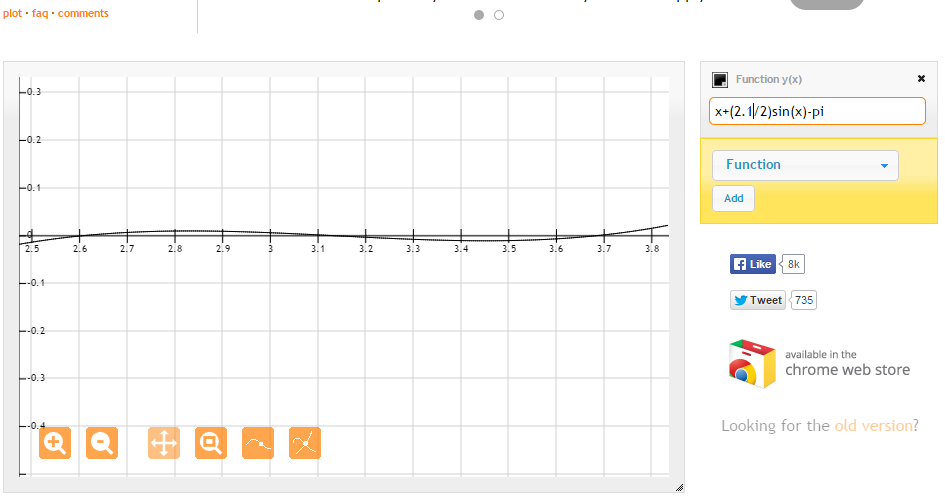It's like a cubic.

- 6 years, 4 months ago

I did this analysis too , well it's very interesting.

- 6 years, 4 months ago

Thanks, the analysis was an interesting read.

Staff - 6 years, 4 months ago

Can you post here the Java code that you've used ?

- 6 years, 4 months ago

  1 2 3 4 5 6 7 8 9 10 11 12 13 14 15 16 17 18 19 20 21 22 23 double d=1 ; int i=1 ; for(int j=990 ; j<1001 ; j=j+1) { for( ; ; i=i+1){ d = d + (1.00+(j/1000.00))*Math.sin(d) ; if(((10000*(d-Math.PI))/(Math.PI))<1 && -1 < ((10000*(d-Math.PI))/(Math.PI)) ) break ; } System.out.print(j+"-"+i+",") ; d=1 ; i=1 ; } 

- 6 years, 4 months ago

Well, The only thing I can add is that your conjecture isn't true. For example, take $\alpha = \dfrac{k\pi - a_0}{sin(a_0)}$. By taking a large enough $k$, we will have $\dfrac{k\pi - a_0}{sin(a_0)} > 2$

- 6 years, 4 months ago

Well you must give me a counter example to my conjecture, and I can't understand what you have written,kindly elaborate.

- 6 years, 4 months ago

Sorry. I just noticed you had a value for $a_0$ aswell. Take $\alpha = \dfrac{\pi - 1}{sin(1)}$. You can check to see that this is greater than $2$. You can find more values by taking $\alpha = \dfrac{k\pi - 1}{sin(1)}$ for a natural number $k$

- 6 years, 4 months ago

Oh, now I realize I have mistaken, I didn't realize this.

- 6 years, 4 months ago

@Ronak Agarwal I think we can now kill this problem.

I'm going to use gradient descent inspired, mostly qualitative approach for proving my arguments. Consider this: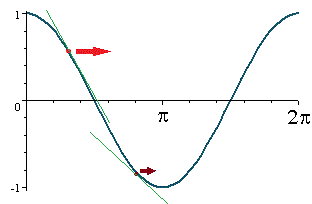This is the $cos(x)$ "attraction basin" that is relevant to this problem. If $\alpha$ is small enough, then, as we know, we end up at $\pi$.

But, if $\alpha>2$, we see that there exists a value $b$ such that $\pi-b=\pi+b+\alpha sin(\pi+b)$ and $\pi+b=\pi-b+\alpha sin(\pi-b)$. (refer comments for proof).

Now, I argue that if if $\alpha>2$, the iterations will start shuttling between the two sides of the basin. Further, a small investigation into the iteration function can show us that it now "attracts" the iterations to the $y=b$ line. If $a_n$ is above it, it is pulled down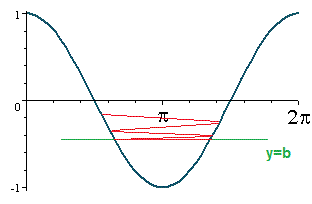If it is below, it is pulled up.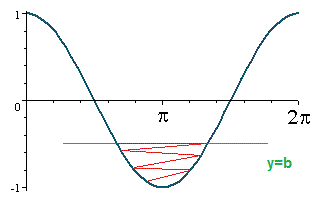The red line represents the motion of the point through successive iterations.

Going even deeper, if $\alpha$ becomes too large as the point escapes from this basin of $cos(x)$ itself, then I am assuming it will diverge(don't have a solid proof for this).

- 6 years, 4 months ago